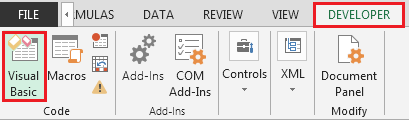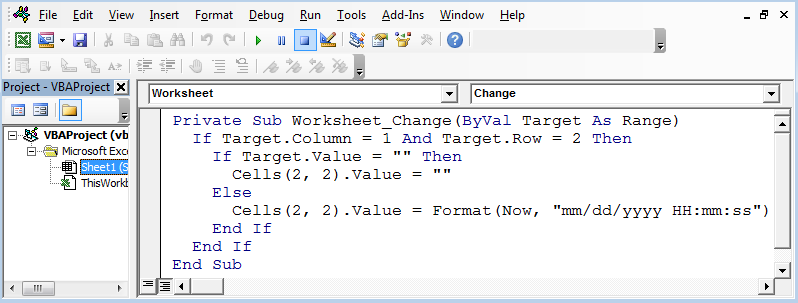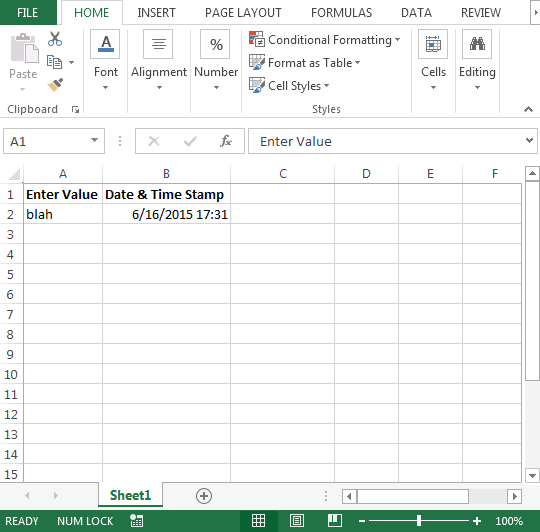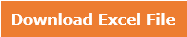Insert Date Time Stamp with VBA

In case you want a VBA code to automatically record date & time stamp in a specific cell & insert date & time stamp based on the changes in other cell, then this article is for you.

In this article, we will learn how to insert Date & Time Stamp, using vba coding.

Question): I want to add date & time stamp in cell B2 through vba code when I enter anything in cell A2.

In order to get date & time stamp, using vba code, we need to follow the below steps to launch VB editor

• Click on Developer tab
• From Code group, select Visual Basic• Copy the below code in Worksheet module

Private Sub Worksheet_Change(ByVal Target As Range)

If Target.Column = 1 And Target.Row = 2 Then

If Target.Value = “” Then

Cells(2, 2).Value = “”

Else

Cells(2, 2).Value = Format(Now, “mm/dd/yyyy HH:mm:ss”)

End If

End If

End Sub• As we enter anything in cell A2; the corresponding cell B2 will get date & time stamp automatically as we are using Worksheet Change event. Refer below snapshot• If we delete value in cell A2 then date & time stamp will also be deleted.

In this way, we can insert date & time stamp using vba in Microsoft Excel 2010.1.